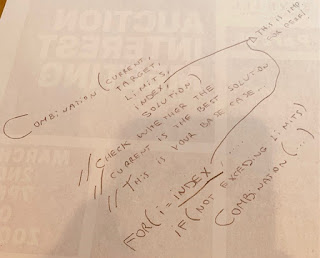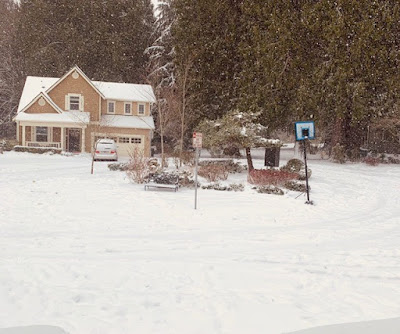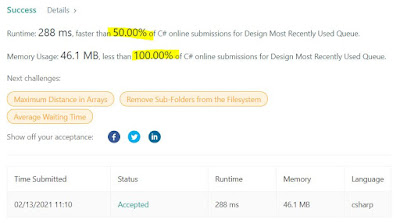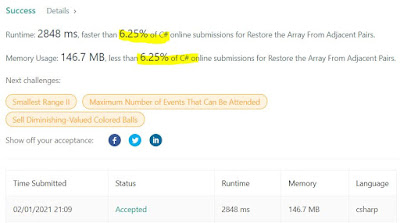## Posts

Showing posts from February, 2021

### General approach to dealing with combinationsThis problem is yet another "combinations" problem, a common LC and interview question, here it is:  Closest Dessert Cost - LeetCode 1774. Closest Dessert Cost Medium 87 13 Add to List Share You would like to make dessert and are preparing to buy the ingredients. You have  n  ice cream base flavors and  m  types of toppings to choose from. You must follow these rules when making your dessert: There must be  exactly one  ice cream base. You can add  one or more  types of topping or have no toppings at all. There are  at most two  of  each type  of topping. You are given three inputs: baseCosts , an integer array of length  n , where each  baseCosts[i]  represents the price of the  i th  ice cream base flavor. toppingCosts , an integer array of length  m , where each  toppingCosts[i]  is the price of  one  of the  i th  topping. target , an integer representing your target price for dessert. You want to make a dessert with a total cost as close to  target  as possible. Return

### A HARD problem to reach 800Decided to pick a HARD problem as problem #800. Here it is:  Unique Paths III - LeetCode 980. Unique Paths III Hard 1292 87 Add to List Share On a 2-dimensional  grid , there are 4 types of squares: 1  represents the starting square.  There is exactly one starting square. 2  represents the ending square.  There is exactly one ending square. 0  represents empty squares we can walk over. -1  represents obstacles that we cannot walk over. Return the number of 4-directional walks from the starting square to the ending square, that  walk over every non-obstacle square exactly once .   Example 1: Input: [[1,0,0,0],[0,0,0,0],[0,0,2,-1]] Output: 2 Explanation: We have the following two paths: 1. (0,0),(0,1),(0,2),(0,3),(1,3),(1,2),(1,1),(1,0),(2,0),(2,1),(2,2) 2. (0,0),(1,0),(2,0),(2,1),(1,1),(0,1),(0,2),(0,3),(1,3),(1,2),(2,2) Example 2: Input: [[1,0,0,0],[0,0,0,0],[0,0,0,2]] Output: 4 Explanation: We have the following four paths: 1. (0,0),(0,1),(0,2),(0,3),(1,3),(1,2),(1,1),(1,0),(2,0

### Nice SubstringEasy LC:  Longest Nice Substring - LeetCode 1763. Longest Nice Substring Easy 35 57 Add to List Share A string  s  is  nice  if, for every letter of the alphabet that  s  contains, it appears  both  in uppercase and lowercase. For example,  "abABB"  is nice because  'A'  and  'a'  appear, and  'B'  and  'b'  appear. However,  "abA"  is not because  'b'  appears, but  'B'  does not. Given a string  s , return  the longest  substring  of  s  that is  nice . If there are multiple, return the substring of the  earliest  occurrence. If there are none, return an empty string .   Example 1: Input: s = "YazaAay" Output: "aAa" Explanation: "aAa" is a nice string because 'A/a' is the only letter of the alphabet in s, and both 'A' and 'a' appear. "aAa" is the longest nice substring. Example 2: Input: s = "Bb" Output: "Bb" Explanation: "B

### Homogenous Substrings and GaussInteresting that this problem actually gets a help from Gauss' formula for summation of consecutive numbers (you know, the one that he taught his teacher when he was only 10... check it out:  Gauss_addition_lesson.pdf (hawaii.edu) ). Here it is:  Count Number of Homogenous Substrings - LeetCode 1759. Count Number of Homogenous Substrings Medium 67 12 Add to List Share Given a string  s , return  the number of  homogenous  substrings of  s .  Since the answer may be too large, return it  modulo   10 9  + 7 . A string is  homogenous  if all the characters of the string are the same. A  substring  is a contiguous sequence of characters within a string.   Example 1: Input: s = "abbcccaa" Output: 13 Explanation: The homogenous substrings are listed as below: "a" appears 3 times. "aa" appears 1 time. "b" appears 2 times. "bb" appears 1 time. "c" appears 3 times. "cc" appears 2 times. "ccc" appears

### Queue using ListNot sure I'd classify this problem as Medium, but in any case here it is:  Design Most Recently Used Queue - LeetCode 1756. Design Most Recently Used Queue Medium 15 0 Add to List Share Design a queue-like data structure that moves the most recently used element to the end of the queue. Implement the  MRUQueue  class: MRUQueue(int n)  constructs the  MRUQueue  with  n  elements:  [1,2,3,...,n] . fetch(int k)  moves the  k th  element  (1-indexed)  to the end of the queue and returns it.   Example 1: Input: ["MRUQueue", "fetch", "fetch", "fetch", "fetch"] [, , , , ] Output: [null, 3, 6, 2, 2] Explanation: MRUQueue mRUQueue = new MRUQueue(8); // Initializes the queue to [1,2,3,4,5,6,7,8]. mRUQueue.fetch(3); // Moves the 3 rd element (3) to the end of the queue to become [1,2,4,5,6,7,8,3] and returns it. mRUQueue.fetch(5); // Moves the 5 th element (6) to the end of the queue to become [1,2,4,5,7,8,3,6] and return

### Graph in disguise